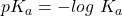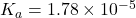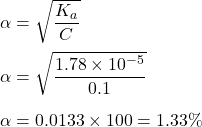## Calculate the % dissociation of 0.10 M solution of acetic acid (pKa = 4.75)

Question

Calculate the % dissociation of 0.10 M solution of acetic acid (pKa = 4.75)

in progress 0
4 weeks 2021-08-18T04:02:06+00:00 1 Answers 1 views 0

1. Given :

Molarity of acetic acid solution, M = 0.10 M.

pKa of acetic acid, pKa = 4.75 .

To Find :

Percentage dissociation of 0.10 M solution of acetic acid.

Solution :

We know,Taking antilog both side, we get :Since, acetic acid has 1 hydrogen atom to loose , so it is a monoprotic acid.

Now, percentage dissociation of monoprotic acid is given by :Hence, this is the required solution.